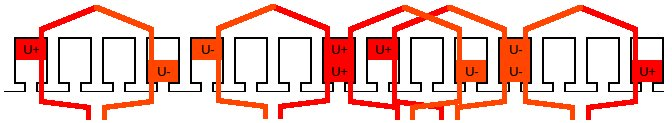# Coil span

The coil span is the distance between one conductor of a certain phase and the corresponding return conductor.

The coil span can be given as a number of slot pitches, as a length, or as an angle.

Example: Below is the coil arrangement of phase U of a 4-pole 15-slot two-layer winding. The fundamental winding factor for this winding arrangement is 0.91.Fig. 1 Double-layer 4-pole 15-slot winding with a coil span of 3 slot pitches.

Assuming that the radius to the middle of the winding slot is $r$ = 100 mm, the coil span can be expressed as:

• 3 slot pitches, corresponds to:
• 72 degrees mechanical $\left(= \frac{3}{15} \cdot 360\: \mathrm{deg} \right)$, corresponds to:
• 144 degrees electrical $\left(= \frac{3}{15} \cdot 360\: \mathrm{deg} \cdot \frac{p}{2} \right)$, corresponds to:
• 125.7 mm $\left(=2\pi \cdot r \cdot \frac{72\: \mathrm{deg}}{360\: \mathrm{deg}} \right)$